One of the best known routing problems is the traveling salesman problem (TSP) which requires a salesman to travel from a starting location to a predetermined set of destinations exactly once and complete the tour by returning to the place of origin.  In doing so, the goal or objective of the salesman is to travel along that route that minimizes the total distance.  Many variations of the TSP have been formulated and used to solve routing problems.  However, the TSP is not directly applicable in the field of reverse logistics.  Powered by environmental and economic motivation some companies are not only responsible for the distribution of products but also for the transportation of reusable products in the reverse direction.  An example of this is the delivery of water, where a truck with a load of full bottles ordered by customers travels a route to not only make deliveries but also pick-up empty bottles that must be returned to the depot.  Not limited to consumable products reverse logistics can also be used in the transportation of people.  A non-profit organization in New York helps to transport children outside the city but the buses have a limited number of seats which means that they must drop off a child and eventually pick-up another child to return home (Ganesh & Narendran, 2008, pp. 1221-1222).  Even though reverse logistical concepts are vastly used in daily operations throughout the world there is little literature related to this field.  The traveling salesman problem with simultaneous delivery and pick-up (TSDP) is an extension of the TSP that focuses on the distribution of commodities and the collection of reusable empty packages.  Like the TSP, the TSDP has a starting location and an objective of minimizing the total distance traveled but the transportation vehicle has a limited capacity and each destination (node) will not only have an order demand but also a return quantity.  TASTE, which stands for Tour construction using Agglomeration and Search for Tour improvement using Enhanced simulated annealing, is a two-phase heuristic to solve a routing problem with simultaneous delivery and pick-up (Ganesh & Narendran, 2008, p. 1221).  In this paper, assumptions from the article TASTE: a two-phase heuristic to solve a routing problem with simultaneous delivery and pick-up will be acknowledged, a mixed integer linear program (MINLP) will be formulated, the TASTE procedure will be established and explained with the use of simple examples, and main conclusions will be discussed.

Before developing and stating any conclusions from the article, it is essential to identify all assumptions that have been made.  In order to conceptualize the TSDP consider a distribution system consisting of a warehouse that acts as a transshipment node, which is a node that can both receive and send goods from other nodes, and many customer locations (Winston & Venkataramanan, 2003, 400).  Each location has a delivery demand and a pick-up demand while the warehouse is the origin and destination of each demand, respectively.  The warehouse is assumed to have the ability to maintain inventory levels of products at customer locations and the ability to make decisions regarding the size of delivery demands and the timing of replenishments for each customer.  Furthermore, it is assumed that the size of the pick-up demands follow a given proportional ratio of the size of the delivery demands.  In regards to the actual routing of the delivery system it is assumed that the route starts and ends at the node of origin, also known as the depot.  Each node  in N, where N = {1, 2, …, n} represents the node set of customer locations with node 0 referring to the node of origin is visited exactly once.  Split delivery, which is a method of ordering a larger quantity to secure a lower price and dividing the delivery into smaller quantities spread out over time in order to control inventory, is not permitted.  The distance from node i to node j is equal to the distance from node j to node i, Cij = Cji for all i and j. Lastly, all delivery quantities are loaded at the depot and all quantities picked up must be unloaded only at the depot (Ganesh & Narendran, 2008, p. 1222).  With the assumptions stated it is now possible to formulate a mixed integer linear program (MINLP) to solve TSDP.

In order to express the TSDP as a MINLP the notation of the problem must be defined.  Let S be the set of customer locations, T be the set of nodes with depot 0; T = [S  {0}], N be the total number of nodes; N = |S|, {n: 1, 2, …, N} represents customer locations with n = 0 denoting the depot, Cij be the cost to travel from node i to node j, di be the delivery request for node i; i = 1, 2, …, N, pi be the pick-up request for node i; i = 1, 2, …, N and Q be the capacity of the vehicle.  The decision variables, which are variables whose values are under our control and influence the performance of the system, are Di which is the load remaining to be delivered by the vehicle when departing from node i, Pi which is the cumulative load picked up by the vehicle when departing from node i and Xij which is equal to one if the vehicle travels directly from i to j and zero otherwise.  It should be noted that the distance matrix Cij satisfies the triangular inequality which means that ||z|| = ||x + y|| < ||x|| + ||y|| (refer to figure 1).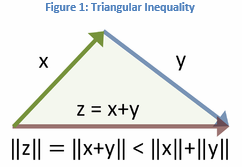Furthermore, at every node in a path the sum of the loads picked up and the quantities remaining to be delivered must be less than or equal to the vehicle capacity.  With the variables and constraints defined the TSDP can be formulated as follows: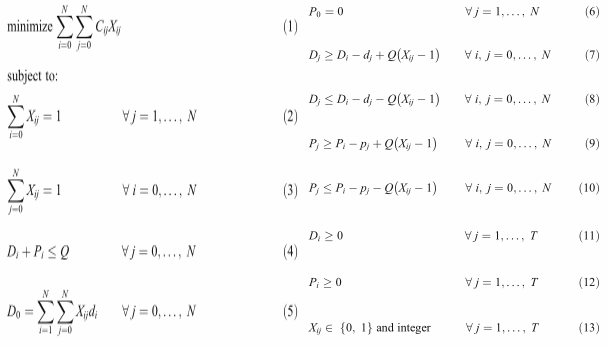The objective function is to minimize the total cost traveled.  Constraints (2) and (3) restrict the visiting of a node to exactly one.  Constraint (4) states that the remaining delivery amount after departing from node i summed with the load picked up at node i must be less than or equal to the vehicle capacity.  Constraint (5) ensures that the total delivery for a tour is loaded on the vehicle.  Constraint (6) states that there is no pick-up at node 0, which is the depot.  Constraints (7) thru (10) decrease the amount remaining to be delivered; increase the amount picked up and eliminates the possibility of sub-tours.  Constraints (11) and (12) are non-negativity conditions and constraint (13) creates the binary condition of whether node i to node j is traveled or not.  Although the TSDP can be formulated as a mixed integer linear program it has been proven that the TSP is NP- complete which means that the TSDP is also NP-complete (Ganesh & Narendran, 2008, 1223).  Being a member of the non-deterministic polynomial (NP) class implies that computational effort can increase rapidly for problems of even moderate sizes which makes seeking an optimal solution impractical.  Hence, the two-phase heuristic procedure TASTE was developed in order to calculate near optimal solutions within a reasonable computational time.

The first phase of TASTE constructs an initial solution using a constructive heuristic (CH) procedure based on the cheapest agglomeration of nodes.  Distance and load-feasibility, which checks to make sure that the vehicle should balance the route with respect to delivery and pick-up capacities, are considered and ensured with the computation of the net load.  The algorithm proceeds as follows: Step one is to calculate the net load N(j) for each node j; N(j) =  (pj – dj).  Note that N(j) is unrestricted.  Step two forms a tour from the depot to all nodes carrying negative net loads.  Then nodes with positive net load are added to the tour such that the distance traveled is minimal.  Once the tour is complete an initial tour, σ0, is created and phase one of TASTE is complete.

To illustrate phase one of TASTE consider the following simple example in figure 2.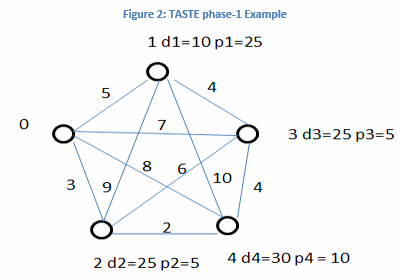The results of the first step, which is to calculate the net load for all nodes, are shown in table 1.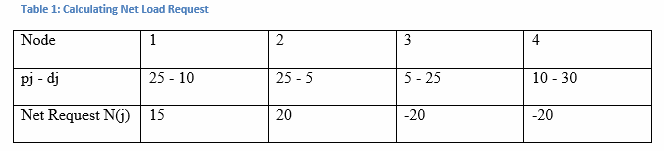Once the net loads have been determined the next step is to create a tour from the depot (node 0) to the nodes with negative net loads.  By traveling to the locations with negative net load, which means that the delivery demand is greater than the pick-up demand, this ensures that the capacity of the vehicle will not be exceeded.  If the vehicle started its tour by traveling to locations with a positive net load, implying that there is a larger pick-up demand than delivery demand, then it is possible that the vehicle capacity could be exceeded.  In the above example two locations have the same negative net load, therefore, to break the tie visit the node that is nearest to the depot first.  Figure 3 depicts the tour with negative net loads.   Lastly to obtain the initial tour, σ0, add positive net load nodes to the tour such that the distance traveled is minimized.  The initial tour for the above example is shown in figure 4.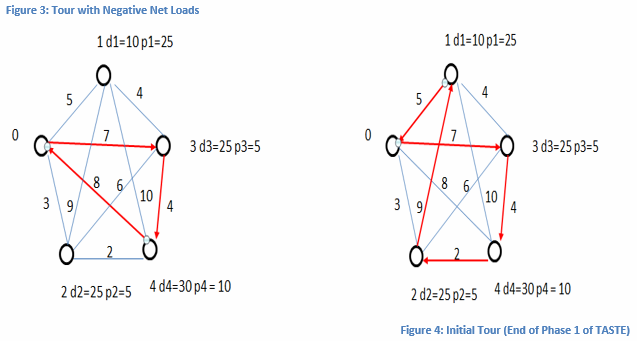Phase two of TASTE is the improvement algorithm that uses meta-heuristics, which is a general solution method that provides both a general structure and strategy guidelines for developing a specific heuristic method to fit a particular kind of problem, because they are the most promising and effective solution methods for the TSP and vehicle routing problems (VRP) (Hillier & Lieberman, 2010, p. 607).  The meta-heuristic implemented in the procedure of TASTE is simulated annealing (SA).  SA is a local search meta-heuristic in the sense that it conducts local searching while guiding the process intelligently, offering the possibility of accepting, in a controlled manner, solutions that do not descend along the path of search (Ganesh & Narendran, 2008, pp. 1224-1225).  The first step of SA is to generate a solution X0 for the problem, which was created at the end of phase one in TASTE.  Arrive at an initial temperature T0, number of iterations at each step I, choose the cooling schedule temperature reduction δ, where 0 ≤ δ ≤ 1, based on experience or preliminary studies and set Tcur = T0, Xcur = X0,

Xbest = Xcur and Zbest = Z(Xcur).  Step two is for count = 1 to I, randomly generate a new solution Xcount.  If          Z(Xcount) is better than Z(Xcur) set Xcur = Xcount, otherwise calculate ΔC = best objective function value to date – current objective function value = Z(Xcount) – Z(Xcur) and set Xcur = Xcount with probability .  On the other hand, if Zbest is worse than Zcur set Xbest = Xcur, Zbest = Zcur and update the count.  The third and final step of SA is to set Tcur = Tcur – δ.  If the stopping criteria of temperature level (for example, temperature < 10) or another stopping criteria is met then stop with Zbest and Xbest as the SA solution, otherwise return to step two with Tcur (Winston & Venkataramanan, 2003, pp.806-807).  Although SA is a good procedure for solving routing problems it imposes a trade-off between computational time and the quality of the solution. Therefore TASTE combines SA with evolutionary computation (EC) to produce enhanced simulated annealing (ESA) which makes each tour determine its appropriate temperature instead of forcing a uniform cooling schedule by using Or-opt exchange, a local search procedure that generates neighborhoods.

The Or-opt algorithm begins by considering an initial route with n vertices and set t = 1 = location of first node in tour and s = 2 = chain of consecutive vertices from position t to t + 2 that must be removed and inserted between all remaining pairs of consecutive vertices on the route. Note that s is an arbitrary value.  If one or more insertions decreases the cost of the route then choose new route, otherwise set t = t + 1, if t ≤ n + 1 repeat the previous step.  Lastly, set t = 1 and s = s-1, if s > 0 go to step two, in which a segment is removed and inserted between all remaining consecutive vertices, otherwise stop (Ganesh & Narendran, 2008, p. 1226).  The Or-opt with two nodes, s = 2, will be shown in figures 5-7.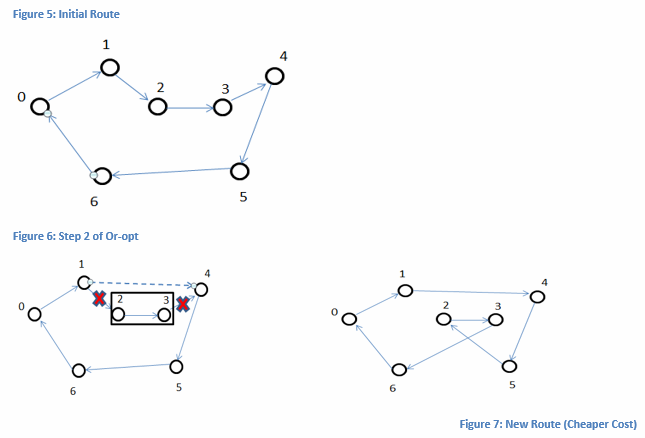With the SA and Or-opt algorithms defined it is now possible to describe the ESA procedure with a clear understanding of each steps purpose.  Step one of ESA is to create an initial solution, which is obtained at the end of phase one of TASTE.  Step two is to set the initial temperature Tmax, cooling rate α (0 ≤ α ≤ 1) and the iteration number I = 0.  Step three uses Or-opt for neighborhood generation.  Step four calculates the objective functions of the newly created routes and the initial route.  Step five ranks the newly created routes in ascending order.  Step six calculates the maximum temperature, Tmax, for each newly created route.  Step seven compares the values of the objective functions for the newly created routes with the objective function value of the initial route using the third step of the SA algorithm previously discussed.  Finally, step eight states to stop if the solution converges or the maximum number of iterations has been reached, otherwise go to step three (Ganesh & Narendran, 2008, p. 1226).  After formulating the TASTE procedure, it was coded in C to analyze the effectiveness and efficiency of the algorithm.

Benchmark problems for the TSDP do not exist so TASTE was tested on the standard data sets (TSPLIB) of symmetric TSPs, which are available on the University of Heidelberg website, derived TSDP data-sets from TSP data-sets, and randomly generated instances.  Along with possessing a reasonable computational time TASTE resulted in an average deviation of 2.32% from the optimal solutions when compared with data-sets published in literature for the classical TSP, 0.43% average deviation with respect to the randomly generated data-sets for the TSDP and 11.98% when compared to derived data-sets from the existing TSP data-sets and used published solution as lower bounds (Ganesh & Narendran, 2008, pp. 1228, 1230).

In this paper the concepts of reverse logistics were discussed then formulated as a linear program.  Due to the time complexity of the linear program, TASTE, a two-phase heuristic for solving routing problems with simultaneous delivery and pick-ups, was developed with in-depth descriptions and simple examples of the algorithms being used.  After comparing TASTE with several systems it is reasonable to state that TASTE is a fast and reliable procedure to obtain near optimal solutions for the TSDP.  The field of reverse logistics is limited with respect to published literature but the work from the article TASTE: a two-phase heuristic to solve a routing problem with simultaneous delivery and pick-up and others leave room for developing new ideas and heuristics in the field of reverse logistics, in particular focusing on methodologies that account for time windows.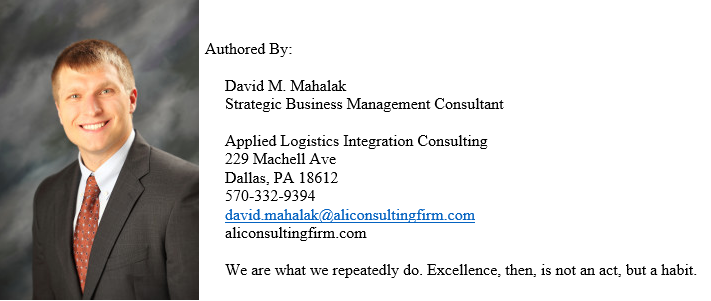References

Ganesh, K., & Narendran, T.T. (2008). “TASTE: A Two-phase Heuristic to Solve a Routing Problem with Simultaneous Delivery and Pick-up.” The International Journal of Advanced Manufacturing Technology, 37. 1221-1231.

Hillier, F.S., & Lieberman, G.J. (2010). Introduction to Operations Research. 9th ed. New York, NY: McGraw-Hill Higher Education.

Winston, W.L, & Venkataramanan, M. (2003). Introduction to Mathematical Programming: Operations Research. 4th ed. Duxbury.